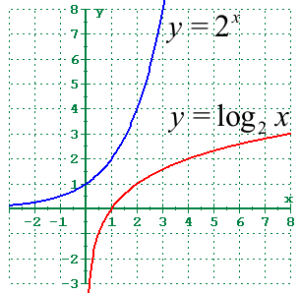# Iterated Logarithm Function: Definition, Examples

Types of Functions > Iterated Logarithm Function

Contents:

## What is a Logarithm Function?

A logarithm function is a function of the form:

f(x) = logbx.

It is the inverse function of the exponential function. In notation, that’s: If y = logbx, then by = x.The logarithmic function logbx is read “log base b of x”.

Sometimes a logarithm function is just written as log x. In those cases the writer thought which base to use was obvious, and we use our knowledge of the situation to determine what the expression means exactly. In the vast majority of cases, if you see “log x”, then assume it’s base 10.

## Logarithm Function Properties

• logb1 = 0 . Since b0 = 1, the exponent any base needs to make 1 is always zero.
• logbb = 0 . Since b1 = b, the exponent any base needs to make itself is always just one.
• logbbx = x . Remember log bas b of x and b to the x power are inverse functions. When inverse functions are applied to each other, the inverse out (essentially cancel each other out).
• If we know that logbx = logby, then we know that x = y.
• If we know that logbx = logax, then we know that a = b.

## Special Bases

The logarithm with base 2 is called the binary logarithm, and it is foundational in much of computer science.

The logarithm with base e (≈ 2.718) is called the natural logarithm and is important in math and physics.

The logarithm with base 10 is the common logarithm, and is used in science, engineering and many other fields.

## Iterated Logarithm Function

The iterated logarithm functionlog * n (“log star of n”) is defined as the smallest natural number k for which
log(i) ≤ 1.
The function is not defined for n < 1.

Formally, it is defined as :

• log * n = 0 if n ≤ 1
• log * n = 1 + log * (log n) if n > 1.

This slow growing function measures how many times you have to take the logarithm of n before it drops to one  or how many times you can take the log n base of a number .

A few examples:

• log * 1 = 0
• log * 2 = 1
• log * 4 = 2
• log * 16 = 3
• log * 65,536 = 4.
• log * (265536) = 5

This function is very slow-growing. Since the number of atoms in the universe if about 1080, which is a lot less than 265536, it’s very rare to see any use the iterated logarithm function for numbers that high: lg * 1020 exceeds the number of bytes in all of the computers on the planet .

Uses include algorithm analysis and building search trees (the Union-Find algorithm) in computer science, probability theory , mathematical systems and control theory , as well as various mathematical areas like the study of sets in bounded systems.

## Iterated Logarithm Function & Tower-of-2

The iterated logarithm function is the inverse of the tower-of-2 function (similar to how log2x is the inverse of 2x). The first few towers-of-2:

• tower-of-2(1) = 2
• tower-of-2(2) = 22 = 4
• tower-of-2(3) = 16
• tower-of-2(4) = 65,536
• tower-of-2(5) ≈ 10006,553

As this is the inverse of the iterated logarithm function, it grows very slowly. If you’re trying to calculate log * n by hand, it’s often much easier to calculate tower-of-2 first, then compare the number in question to see which “bucket” it falls into .

## Iterated Logarithm Function: References

 600.363 Introduction to Algorithms / 600.463 Algorithms I
 Problem Set 1: RMQ.
 Disjoint Set Data Structure.
 Eichelsbacher, P. & Lowe, M. Workshop on Probability Theory and Its Applications. Retrieved July 31, 2021 from: https://citeseerx.ist.psu.edu/viewdoc/download?doi=10.1.1.30.7719&rep=rep1&type=pdf
 Blondel, V. & Megretski, A. [Eds.]. Unsolved Problems in Mathematical Systems and Control Theory. Retrieved July 31, 2021 from: https://citeseerx.ist.psu.edu/viewdoc/download?doi=10.1.1.134.1462&rep=rep1&type=pdf
 Elkins, D. (2017). Computing the Iterated Logarithm (log-star) by hand. Retrieved July 31, 2021 from: https://math.stackexchange.com/questions/2553258/computing-the-iterated-logarithm-log-star-by-hand

CITE THIS AS:
Stephanie Glen. "Iterated Logarithm Function: Definition, Examples" From StatisticsHowTo.com: Elementary Statistics for the rest of us! https://www.statisticshowto.com/iterated-logarithm-function/